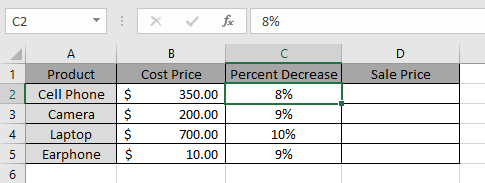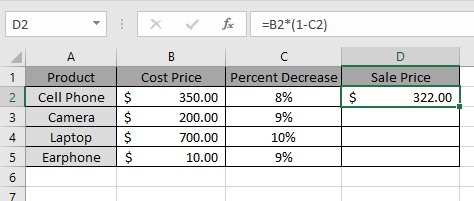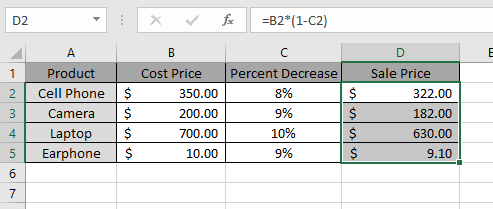# Mathematical Percentage Decrease Change in Excel

In this article, how to use percentage decrease change formula in Excel 2016.
We need to find the percentage decrement in value due to decrement percentage change.
Generic formula

=Value * (1 - percentage)

Value : current value
Percentage : decrease percentage change
Note: It returns a #VALUE! Error if values are not provided with the correct format.
Let’s take an example to understand it.Here we have some products with their current Cost price and percentage decrement.
And we need to find out the new discounted Price for the products.
Use the formula

=B2*(1-C2)

B2 : Current Value
C2 : percentage change in Value
Explanation :
Value gets multiplied with (1 - C2) to get the dicounted amount.Here the new Sales Price is listed in the Sales Price column.
Copy the formula to other cells using shortcut key Ctrl + DThis method can be used for various purposes. As you can see here the new Sales Price is listed in Sales Price column.
Hope you understood How to get the percentage decrease change and its how to use it in Excel 2016. Find more articles on Mathematical formulation here. Please share your query below in the comment box. We will assist you.

Popular Articles :

50 Excel Shortcut to Increase Your Productivity : Get faster at your task. These 50 shortcuts will make you work even faster on Excel.

How to use the VLOOKUP Function in Excel : This is one of the most used and popular functions of excel that is used to lookup value from different ranges and sheets.

How to use the COUNTIF function in Excel : Count values with conditions using this amazing function. You don't need to filter your data to count specific values. Countif function is essential to prepare your dashboard.

How to use the SUMIF Function in Excel : This is another dashboard essential function. This helps you sum up values on specific conditions.

Terms and Conditions of use

The applications/code on this site are distributed as is and without warranties or liability. In no event shall the owner of the copyrights, or the authors of the applications/code be liable for any loss of profit, any problems or any damage resulting from the use or evaluation of the applications/code.